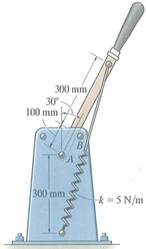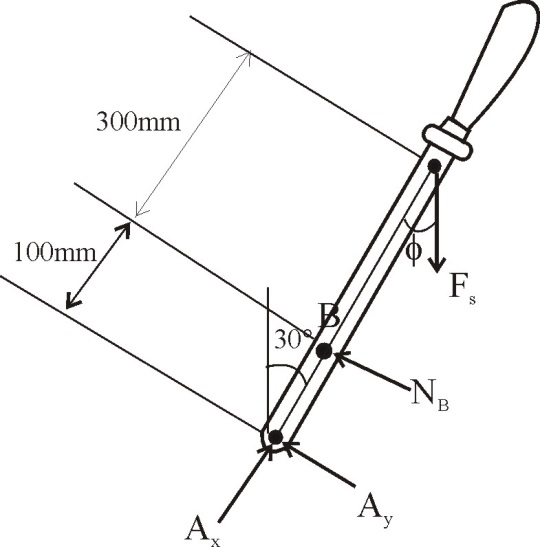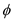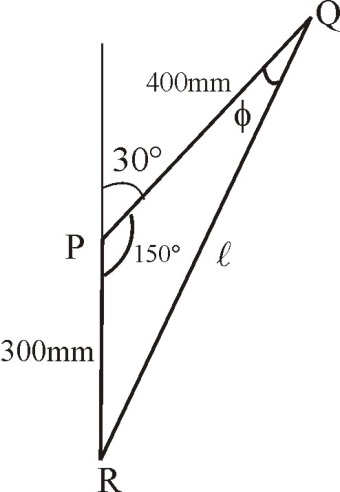Problem

# The toggle switch consists of a cocking lever that is pinned to a fixed frame at A and hel...

The toggle switch consists of a cocking lever that is pinned to a fixed frame at A and held in place by the spring which has an unstretched length of 200 mm. Determine the magnitude of the resultant force at A and the normal force on the peg at B when the lever is in the position shown.#### Step-by-Step Solution

Solution 1

Draw the free body diagram of the lever.Consider triangle PQR and calculate the length of the spring and the angle.Calculate the length of the spring by applying cosine rule to the triangle $$P Q R$$.

$$l^{2}=300^{2}+400^{2}-2 \times 300 \times 400 \cos 150^{\circ}$$

$$l=\sqrt{300^{2}+400^{2}-2 \times 300 \times 400 \cos 150^{\circ}}$$

$$=676.64 \mathrm{~mm}$$

Calculate the angle $$\phi$$ by applying sine rule to the triangle $$\mathrm{PQR}$$.

$$\frac{\sin 150}{l}=\frac{\sin \phi}{300}$$

Substitute $$676.64 \mathrm{~mm}$$ for $$l$$.

$$\frac{\sin 150^{\circ}}{676.64}=\frac{\sin \phi}{300}$$

$$\sin \phi=\frac{300}{676.64} \sin 150^{\circ}$$

$$\phi=12.8^{\circ}$$

Calculate the spring force by using the equation,

$$F_{S}=K(l-\delta) \ldots \ldots(1)$$

Here, $$F_{S}$$ is the spring force, $$K$$ is the stiffness of the spring, $$l$$ is the length of the spring and $$\delta$$ is the unstretched length of the spring.

Substitute $$5 \mathrm{~N} / \mathrm{m}$$ for $$K, 0.67664 \mathrm{~m}$$ for $$l$$ and $$0.2 \mathrm{~m}$$ for $$\delta$$.

\begin{aligned} F_{S} &=5 \times(0.67664-0.2) \\ &=2.38 \mathrm{~N} \end{aligned}

Calculate the algebraic sum of the moments of all the forces about point $$A .$$

$$\left(N_{B} \times 100\right)-\left(F_{s} \sin \phi \times 400\right)=0$$

Substitute $$2.38 \mathrm{~N}$$ for $$F_{s}$$ and $$12.8^{\circ}$$ for $$\phi$$.

$$\left(N_{B} \times 100\right)-\left(2.38 \sin 12.8^{\circ} \times 400\right)=0$$

$$N_{B}=\frac{\left(2.38 \sin 12.8^{\circ} \times 400\right)}{100}$$

$$=2.11 \mathrm{~N}$$

Therefore, the normal force on the peg at $$B$$ is $$N_{B}=2.11 \mathrm{~N}$$.

Apply equilibrium equation to the lever along the horizontal direction.

$$\sum F_{x}=0$$

$$A_{x}-F_{s} \cos \phi=0$$

Substitute $$2.38 \mathrm{~N}$$ for $$F_{s}$$ and $$12.8^{\circ}$$ for $$\phi$$.

$$A_{x}-F_{s} \cos \phi=0$$

\begin{aligned} A_{x} &=F_{S} \cos \phi \\ &=2.38 \cos 12.8^{\circ} \\ &=2.32 \mathrm{~N} \end{aligned}

Apply equilibrium equation to the lever along the vertical direction.

$$\sum F_{y}=0$$

$$A_{y}+N_{B}-F_{S} \sin \phi=0$$

Substitute $$2.38 \mathrm{~N}$$ for $$F_{s}, 2.11 \mathrm{~N}$$ for $$N_{B}$$ and $$12.8^{\circ}$$ for $$\phi$$.

$$A_{y}+N_{B}-F_{S} \sin \phi=0$$

\begin{aligned} A_{y} &=F_{s} \sin \phi-N_{B} \\ &=2.38 \sin 12.8^{\circ}-2.11 \\ &=-1.58 \mathrm{~N} \end{aligned}

Calculate the resultant force at $$\mathrm{A}$$ by using the following equation:

$$F_{A}=\sqrt{A_{x}^{2}+A_{y}^{2}}$$

Here, $$F_{A}$$ is the resultant force at $$\mathrm{A}$$.

Substitute $$2.32 \mathrm{~N}$$ for $$A_{x}$$ and $$-1.58 \mathrm{~N}$$ for $$A_{y}$$.

\begin{aligned} F_{A} &=\sqrt{2.32^{2}+(-1.58)^{2}} \\ &=2.81 \mathrm{~N} \end{aligned}

Therefore, the resultant force at $$\mathrm{A}$$ is $$F_{A}=2.81 \mathrm{~N}$$.